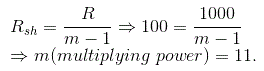# MCQs on Electrical And Electronics Measurements

##### Page 3 of 19. Go to page 1 2 3 4 5 6 7 8 9 10 11 12 13 14 15 16 17 18 19
01․ Unit of deflection sensitivity of a CRO is
V / mm.
meter / volt.
mm per mV.
mm / V.

The deflection sensitivity is actually the voltage required to transfer the electronic beam through a unit of length. So, the unit of deflection sensitivity is meter/volt.

02․ Swamping resistance is used to compensate
error due to temperature true variations.
error due to strong magnetic field.
both A and B.
none of these.

Swamping resistance is connected in series with the meter and that resistance has a negligible resistance co-efficient in order to reduce temperature errors in shunted ammeters. It is generally made of manganin.

03․ The chemical effect of current is used in
D.C ampere hour meter.
D.C ammeter.
D.C energy meter.
none of the above.

The battery is rated in terms of ampere hour. The chemical effect of current is created by the batteries. Hence, the DC ampere hour meter is used to measure the ampere hour rating of the battery.

04․ A galvanometer with a full scale current of 10 mA has a resistance of 1000 Ω. The multiplying power (the ratio of measured current to galvanometer current) of 100 Ω shunt with this galvanometer is
110.
100.
11.
10.05․ The high torque to weight ratio in an analog indicating instrument indicates
low friction loss.
high friction loss.
nothing related to friction loss.
none of these.

The high torque to weight ratio in an analog indicating instrument always indicates a fast response of the system. Hence, the system has low friction loss.

06․ Which of following is a vector quantity?
Magnetic field intensity.
Flux density.
Magnetic potential.
Relative permeability.

Magnetic field intensity is a force. The vector notation should be required to indicate the magnitude of intensity and the direction of force which is developed by that intensity. Hence, the magnetic field intensity is a vector quantity from the above explanation.

07․ A 0 - 250V voltmeter has a guaranteed accuracy of 2% of full scale reading. What is the percentage of error if it measures 150V?
2%
3.33%
5%
1%

Maximum error = 2% of 250 = 5V; Percentage error when it measures 150V = 5 × 100/150 = 3.33%.

08․ A spring-controlled moving iron voltmeter draws a current of 1 mA for full scale value of 100V. If it draws a current of 0.5 mA, the meter reading is
25 V.
50 V.
100 V.
200 V.

09․ The inductance of a certain moving iron ammeter is expressed as L = 2+5θ-θ2 µH. The control spring torque is 2 × 10-6 Nm/radian. Find the deflection in radians for a current of 2A.
1
2
4
5

The deflecting torque of a moving iron instrument (T) = 0.5I2dL/dθ. I = 2A; T = 2 × 10-6 Nm/radian; dL/dθ = 5-2θ µH; So, 5-2θ = 1; θ = 2.

10․ The internal resistance of a milli-ammeter must be very low for
high sensitivity.
high accuracy.
high precision.
minimum effect on the current in the circuit.

A high value of internal resistance will vary the current considerably in a milli-ammeter as the current is in milli range. So the internal resistance of a milli-ammeter must be very low.

<<<12345>>>